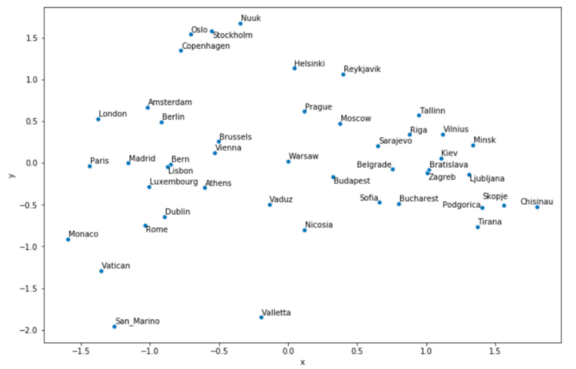使用Python可视化Word2vec的结果

2020/09/29 23:55

Word2vec绝对是我在自然语言处理研究中遇到的最有趣的概念。想象一下，有一种算法可以成功地模拟理解单词的含义及其在语言中的功能，它可以在不同的主题内来衡量单词之间的接近程度。

• 从广义上讨论word2vec理论；

• 下载原始的预训练向量；

• 看看一些有趣的应用程序：比如对一些单词进行算术运算，比如著名的king-man+woman=queen等式

• 根据word2vec向量看看我们能多精确地来绘制欧洲的首都。

word2vec的原始研究论文和预训练模型来自2013年，考虑到NLP文献的扩展速度，目前它是老技术。较新的方法包括GloVe（更快，可以在较小的语料库上训练）和fastText（能够处理字符级的n-gram）。

Quick Word2Vec简介

Word2vec是一个词嵌入过程。这个概念相对简单：通过一个句子一个句子地在语料库中循环去拟合一个模型，根据预先定义的窗口中的相邻单词预测当前单词。

word2vec中有两种建模方法：skip-gram和continuous bag of words，这两种方法都有各自的优点和对某些超参数的敏感性……但是你知道吗？我们将不拟合我们自己的模型，所以我不会花时间在它上面。

如何下载和安装

from gensim.models.keyedvectors import KeyedVectors

binary = True, limit = 1000000)

limit参数定义了要导入的单词数，100万对于我来说已经足够了。

探索Word2vec

word_vectors['dog']

word_vectors.most_similar(positive = ['nice'], topn = 5)

[('good', 0.6836092472076416),
('lovely', 0.6676311492919922),
('neat', 0.6616737246513367),
('fantastic', 0.6569241285324097),
('wonderful', 0.6561347246170044)]

[('J.Gordon_###-###', 0.38660115003585815),
('M.Kenseth_###-###', 0.35581791400909424),
('D.Earnhardt_Jr._###-###', 0.34227001667022705),
('G.Biffle_###-###', 0.3420777916908264),
('HuMax_TAC_TM', 0.3141660690307617)]

word_vectors.doesnt_match(
['Hitler', 'Churchill', 'Stalin', 'Beethoven'])

word_vectors.most_similar(
positive = ['father', 'woman'], negative = ['man'], topn = 1)

[('mother', 0.8462507128715515)]

word_vectors.most_similar(
positive = ['doctor', 'woman'], negative = ['man'], topn = 1)

[('gynecologist', 0.7093892097473145)]

Map函数

1. 找到列表中每个单词的词向量表示；

2. 利用主成分分析法将维数降到2；

3. 创建散点图，将单词作为每个数据点的标签；

4. 另外一个额外的好处是，可以从任何维度“旋转”结果——主成分分析的向量是任意方向的，当我们绘制地理单词时，我们可能想要改变这个方向，看是否可以与现实世界的方向一致。

import matplotlib.pyplot as plt
import seaborn as sns
from sklearn.decomposition import PCA

def plot_2d_representation_of_words(
word_list,
word_vectors,
flip_x_axis = False,
flip_y_axis = False,
label_x_axis = "x",
label_y_axis = "y",
label_label = "city"):

pca = PCA(n_components = 2)

word_plus_coordinates=[]

for word in word_list:

current_row = []
current_row.append(word)
current_row.extend(word_vectors[word])
word_plus_coordinates.append(current_row)

word_plus_coordinates = pd.DataFrame(word_plus_coordinates)

coordinates_2d = pca.fit_transform(
word_plus_coordinates.iloc[:,1:300])
coordinates_2d = pd.DataFrame(
coordinates_2d, columns=[label_x_axis, label_y_axis])
coordinates_2d[label_label] = word_plus_coordinates.iloc[:,0]
if flip_x_axis:
coordinates_2d[label_x_axis] = \
coordinates_2d[label_x_axis] * (-1)
if flip_y_axis:
coordinates_2d[label_y_axis] = \
coordinates_2d[label_y_axis] * (-1)

plt.figure(figsize = (15,10))
p1=sns.scatterplot(
data=coordinates_2d, x=label_x_axis, y=label_y_axis)

x = coordinates_2d[label_x_axis]
y = coordinates_2d[label_y_axis]
label = coordinates_2d[label_label]

texts = [plt.text(x[i], y[i], label[i]) for i in range(len(x))]

capitals = [
'Bratislava', 'Brussels', 'Bucharest', 'Budapest',
'Chisinau', 'Copenhagen','Dublin', 'Helsinki', 'Kiev',
'Minsk', 'Monaco', 'Moscow', 'Nicosia', 'Nuuk', 'Oslo',
'Paris','Podgorica', 'Prague', 'Reykjavik', 'Riga',
'Rome', 'San_Marino', 'Sarajevo','Skopje', 'Sofia',
'Vatican', 'Vienna', 'Vilnius', 'Warsaw', 'Zagreb']

plot_2d_representation_of_words(
word_list = capitals,
word_vectors = word_vectors,
flip_y_axis = True)

（翻转y轴是为了创建更像真实贴图的表示。）• 谷歌的一组研究人员训练了一个庞大的神经网络，根据上下文预测单词；

• 他们将每个单词的权重保存在一个300维的向量表示中；

• 我们计算欧洲各国首都的向量；

• 利用主成分分析法将维数降到2；

• 把计算出的成分放在图表上。

参考引用

sklearn机器学习中文官方文档： http://sklearn123.com/

1
3 收藏

作者的其它热门文章0 评论
3 收藏
1